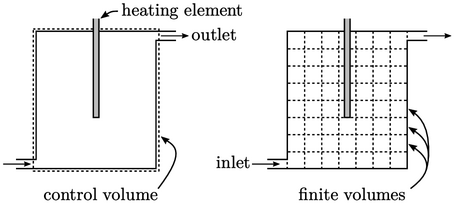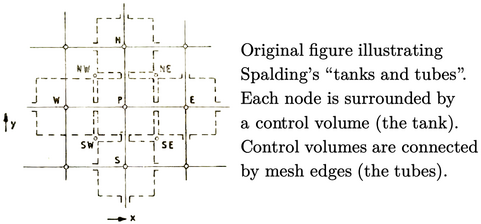## 3.1The ﬁnite volume concept

There are many numerical methods which can be used to solve the partial diﬀerential equations encountered in ﬂuid dynamics. No single method is unequivocally better than others. Rather, the eﬃcacy of each method depends on aspects of the case being simulated, e.g. its size, the required level of accuracy and the characteristics and complexity of the equations being solved.

For a method to be useful, it needs to be programmed into software. Complex programs require good design and design relies on good concepts. So inevitably the concepts behind any method are as important as the details contained within.

The ﬁnite volume method adopts the idea of control volumes used to create models of physical systems. A control volume represents a region of space, which is generally ﬁxed, enclosed by a surface through which ﬂuid ﬂows in and out.It applies conservation equations, e.g. of mass, momentum and energy, by balancing ﬂuxes, due to inﬂow and outﬂow at the bounding surface, with additional sources within the volume. Rather than using a single control volume to describe an entire physical system, e.g. a heating tank, the ﬁnite volume method splits the system domain, i.e. the tank, into multiple connected ﬁnite volumes. Conservation equations are applied to each volume, ensuring that the ﬂuxes of mass, momentum and heat across surfaces are consistent between the volumes they connect.

The perceived wisdom is that the ﬁnite volume method was ﬁrst introduced in the early 1970’s. But the PhD thesis of Runchal1 from 1969 describes a method which is clearly ﬁnite volume. He also published the ﬁgure reproduced below,2 which displays the computational mesh as a set of connected control volumes.Runchal credits the idea to his PhD supervisor Brian Spalding who provided an analogy of tanks connected by tubes in 1967. It captures the essence of ﬁnite volume, which uses a mesh to deﬁne a physical system of control volumes. Its emphasis is on calculating ﬂuxes between volumes and ensuring conservation.

By contrast, other methods, e.g. ﬁnite element, use the mesh to construct mathematical functions to calculate distributions of properties. The ﬁnite volume method does not do that.

1Akshai Runchal, Transfer processes in steady two-dimensional separated ﬂows, PhD Thesis, 1969.
2Akshai Runchal, Brian Spalding and Micha Wolfshtein, Numerical solution of the elliptic equations for transport of vorticity, heat, and matter in two-dimensional ﬂow, 1969.

Notes on CFD: General Principles - 3.1 The ﬁnite volume concept